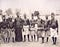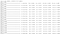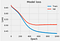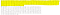# Build Your Own Artificial Neural Network Using Python

In this article I will show you how to create your very own Artificial Neural Network (ANN) using Python ! We will use the Pima-Indian-Diabetes data set to predict if a person has diabetes or not using Neural Networks.

The Pima are a group of Native Americans living in an area consisting of what is now central and southern Arizona. The Pima have the highest reported prevalence of diabetes of any population in the world, and have contributed to numerous scientific gains through their willingness to participate in the research process. Their involvement has led to significant findings with regard to the epidemiology, physiology, clinical assessment, and genetics of both type 2 diabetes and obesity. — National Center for Biotechnology Information“Pima Indians from Arizona. Chief Blue Wing and family.” Department of Anthropology, 1904 World’s Fair

Artificial neural networks (ANN) are computing systems that are inspired by the biological neural networks that constitute the brain. Such systems “learn” to perform tasks by examples, generally without being programmed with any task-specific rules. — Wikipedia

If you prefer not to read this article and would like a video representation of it, you can check out the video below. It goes through everything in this article with a little more detail, and will help make it easy for you to start programming your own Artificial Neural Network (ANN) model even if you don’t have the programming language Python installed on your computer. Or you can use both the video and this article as supplementary materials for learning about ANN’s !

# Programming

The first thing that I like to do before writing a single line of code is to put in a description in comments of what the code does. This way I can look back on my code and know exactly what it does.

`#Description: This program detects/predicts if a person has diabetes (1) or not (0)`

`#Load librariesfrom keras.models import Sequentialfrom keras.layers import Denseimport pandas as pdfrom sklearn.model_selection import train_test_splitimport matplotlib.pyplot as pltplt.style.use('fivethirtyeight')`

Load and store the data set into variable `df`, then print the first 7 rows of data.

`#Store the data setdf = pd.read_csv('diabetes.csv')#Look at first 7 rows of datadf.head(7)`

Show the number of rows and columns in the data set.

`#Show the shape (number of rows & columns)df.shape`

Check for duplicates and remove them.

`#Checking for duplicates and removing themdf.drop_duplicates(inplace = True)`

Show the new shape (if any duplicates) of the data set.

`#Show the shape to see if any rows were dropped df.shape`

Show any missing data in the columns.

`#Show the number of missing (NAN, NaN, na) data for each columndf.isnull().sum()`

Convert the data into an array and print it. This will help the neural network.

`#Convert the data into an arraydataset = df.valuesdataset`

Split the data into an independent / feature data set `X` and a dependent / target data set `y`.

`# Get all of the rows from the first eight columns of the datasetX = dataset[:,0:8] # Get all of the rows from the last columny = dataset[:,8] `

Process the feature data set to contain values between 0 and 1 inclusive, by using the min-max scaler method, and print the values.

`from sklearn import preprocessingmin_max_scaler = preprocessing.MinMaxScaler()X_scale = min_max_scaler.fit_transform(X)X_scale`

Split the data again but this time into 80% training and 20% testing data.

`X_train, X_test, y_train, y_test = train_test_split(X_scale, y, test_size=0.2, random_state = 4)`

Finally we can start building the artificial neural network. The models architecture will contain three layers. The first layer will have 12 neurons and use the ReLu activation function, the second layer will have 15 neurons and use the ReLu activation function, and the third and final layer will use 1 neuron and the sigmoid activation function.

`model = Sequential([    Dense(12, activation='relu', input_shape=( 8 ,)),    Dense(15, activation='relu'),    Dense(1, activation='sigmoid')])`

Compile the model and give it the ‘binary_crossentropy’ loss function (Used for binary classification) to measure how well the model did on training, and then give it the Stochastic Gradient Descent ‘sgd’ optimizer to improve upon the loss. Also I want to measure the accuracy of the model so add ‘accuracy’ to the metrics.

`model.compile(optimizer='sgd',              loss='binary_crossentropy',              metrics=['accuracy'])`

Train the model by using the fit method on the training data, and train it in batch sizes of 57, with 1000 epochs. Give the model validation data to see how well the model is performing by splitting the training data into 20% validation.

Batch: Total number of training examples present in a single batch

Epoch:The number of iterations when an ENTIRE dataset is passed forward and backward through the neural network only ONCE.

Fit: Another word for train

`hist = model.fit(X_train, y_train,          batch_size=57, epochs=1000, validation_split=0.2)`Sample of training on the data up to 15 epochs out of 1000

Visualize how well the model performed, by using graphs ! First visualize the models loss.

`#visualize the training loss and the validation loss to see if the model is overfittingplt.plot(hist.history['loss'])plt.plot(hist.history['val_loss'])plt.title('Model loss')plt.ylabel('Loss')plt.xlabel('Epoch')plt.legend(['Train', 'Val'], loc='upper right')plt.show()`The model loss decreased significantly on both the training and validation data before about 400 epochs.

Now visualize the models accuracy for both the training and validation data.

`#visualize the training accuracy and the validation accuracy to see if the model is overfittingplt.plot(hist.history['acc'])plt.plot(hist.history['val_acc'])plt.title('Model accuracy')plt.ylabel('Accuracy')plt.xlabel('Epoch')plt.legend(['Train', 'Val'], loc='lower right')plt.show()`

Use the model to make a prediction using the testing data set `X_test`
Since neural networks only give probabilities (values between 0 and 1 inclusive), I’ve created a threshold where values .5 and above classify the target data as (1) and values less then that as (0).

I will also print out the actual values of the test set to compare the results.

`#Make a prediction & print the actual valuesprediction = model.predict(X_test)prediction  = [1 if y>=0.5 else 0 for y in prediction] #Thresholdprint(prediction)print(y_test)`Highlighted is the predicted values, and not highlighted is the actual values.

Evaluate the model on the training data set.

`from sklearn.metrics import classification_report,confusion_matrix, accuracy_scorepred = model.predict(X_train)pred  = [1 if y>=0.5 else 0 for y in pred] #Thresholdprint(classification_report(y_train ,pred ))print('Confusion Matrix: \n',confusion_matrix(y_train,pred))print()print('Accuracy: ', accuracy_score(y_train,pred))print()`

Evaluate the model on the testing data set.

`from sklearn.metrics import classification_report,confusion_matrix, accuracy_scorepred = model.predict(X_test)pred  = [1 if y>=0.5 else 0 for y in pred] #Thresholdprint(classification_report(y_test ,pred ))print('Confusion Matrix: \n',confusion_matrix(y_test,pred))print()print('Accuracy: ', accuracy_score(y_test,pred))print()`

Here is another way to get the accuracy on the test data set.

`model.evaluate(X_test, y_test)`

The model accurately identified people as having diabetes or not with 75.9 % accuracy on the test data !

# Conclusion and Resources

That is it, you are done creating your artificial neural network program to detect diabetes !

Again, if you want, you can watch and listen to me explain all of the code in my YouTube video.

If you are interested in reading about machine learning to immediately get started with problems and examples, I recommend you read Hands-On Machine Learning with Scikit-Learn and TensorFlow: Concepts, Tools, and Techniques to Build Intelligent Systems.

It is a great book for helping beginners learn to write machine-learning programs and understanding machine-learning concepts.

Hands-On Machine Learning with Scikit-Learn and TensorFlow: Concepts, Tools, and Techniques to Build Intelligent Systems

# Other Resources

National Center for Biotechnology Information

Written by